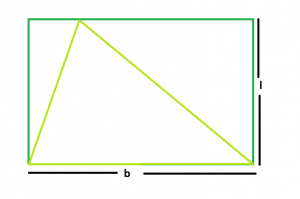# Area of largest triangle that can be inscribed within a rectangle

Given a rectangle of lengthand breadth. The task is to find the area of the biggest triangle that can be inscribed in it.

Examples:

```Input: L = 5, B = 4
Output: 10

Input: L = 3, B = 2
Output: 3
```

## Recommended: Please try your approach on {IDE} first, before moving on to the solution.From the figure, it is clear that the largest triangle that can be inscribed in the rectangle, should stand on the same base & has height raising between the same parallel sides of the rectangle.

So, the base of the triangle = B
Height of the triangle = L

Therefore Area,

```A = (L*B)/2
```

Note: It should also be clear that if base of the triangle = diagonal of rectangle, still the area of triangle so obtained = lb/2 as diagonal of a rectangle divides it into 2 triangles of equal area.

Below is the implementation of the above approach:

## C++

 `// C++ Program to find the biggest triangle ` `// which can be inscribed within the rectangle ` `#include ` `using` `namespace` `std; ` ` `  `// Function to find the area ` `// of the triangle ` `float` `trianglearea(``float` `l, ``float` `b) ` `{ ` ` `  `    ``// a and b cannot be negative ` `    ``if` `(l < 0 || b < 0) ` `        ``return` `-1; ` ` `  `    ``// area of the triangle ` `    ``float` `area = (l * b) / 2; ` `    ``return` `area; ` `} ` ` `  `// Driver code ` `int` `main() ` `{ ` `    ``float` `l = 5, b = 4; ` `    ``cout << trianglearea(l, b) << endl; ` `    ``return` `0; ` `} `

## Java

 `// Java Program to find the biggest triangle ` `// which can be inscribed within the rectangle ` `import` `java.util.*; ` ` `  `class` `GFG ` `{ ` `    ``// Function to find the area ` `    ``// of the triangle ` `    ``static` `float` `trianglearea(``float` `l, ``float` `b) ` `    ``{ ` `     `  `        ``// a and b cannot be negative ` `        ``if` `(l < ``0` `|| b < ``0``) ` `            ``return` `-``1``; ` `     `  `        ``// area of the triangle ` `        ``float` `area = (l * b) / ``2``; ` `        ``return` `area; ` `    ``} ` `     `  `    ``// Driver code ` `    ``public` `static` `void` `main(String args[]) ` `    ``{ ` `        ``float` `l = ``5``, b = ``4``; ` `         `  `        ``System.out.println(trianglearea(l, b)); ` `    ``} ` `} `

## Python3

 `# Python3 Program to find the  ` `# biggest triangle which can be  ` `# inscribed within the rectangle  ` ` `  `# Function to find the area  ` `# of the triangle  ` `def` `trianglearea(l, b) :  ` ` `  `    ``# a and b cannot be negative  ` `    ``if` `(l < ``0` `or` `b < ``0``) : ` `        ``return` `-``1` ` `  `    ``# area of the triangle  ` `    ``area ``=` `(l ``*` `b) ``/` `2` `    ``return` `area ` ` `  `# Driver code  ` `l ``=` `5` `b ``=` `4` `print``(trianglearea(l, b)) ` ` `  `# This code is contributed  ` `# by Yatin Gupta  `

## C#

 `// C# Program to find the biggest  ` `// triangle which can be inscribed ` `// within the rectangle ` `using` `System; ` ` `  `class` `GFG ` `{ ` `// Function to find the area ` `// of the triangle ` `static` `float` `trianglearea(``float` `l, ` `                          ``float` `b) ` `{ ` ` `  `    ``// a and b cannot be negative ` `    ``if` `(l < 0 || b < 0) ` `        ``return` `-1; ` ` `  `    ``// area of the triangle ` `    ``float` `area = (l * b) / 2; ` `    ``return` `area; ` `} ` ` `  `// Driver code ` `public` `static` `void` `Main() ` `{ ` `    ``float` `l = 5, b = 4; ` `     `  `    ``Console.WriteLine(trianglearea(l, b)); ` `} ` `} ` ` `  `// This code is contributed  ` `// by inder_verma `

## PHP

 ` `

Output:

```10
```

GeeksforGeeks has prepared a complete interview preparation course with premium videos, theory, practice problems, TA support and many more features. Please refer Placement 100 for details

My Personal Notes arrow_drop_upCheck out this Author's contributed articles.

If you like GeeksforGeeks and would like to contribute, you can also write an article using contribute.geeksforgeeks.org or mail your article to contribute@geeksforgeeks.org. See your article appearing on the GeeksforGeeks main page and help other Geeks.

Please Improve this article if you find anything incorrect by clicking on the "Improve Article" button below.

Improved By : inderDuMCA, YatinGupta